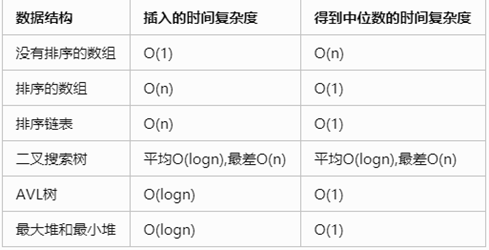### 数据流中的中位数```//排序的数组
const arr=[]
function Insert(num)
{
// write code here
arr.push(num)
for(let i=arr.length-2;arr[i]>num;i--){
[arr[i],arr[i+1]]=[arr[i+1],arr[i]]
}
}
function GetMedian(){
// write code here
if(arr.length&1===1){
return arr[(arr.length-1)/2]
}
return arr[arr.length/2]/2+arr[arr.length/2-1]/2
}```
• 发表于 2020-04-08 01:27
• 阅读 ( 131 )
• 分类：网络文章

## 作家榜 »

1.小编 文章